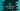# C++ program to find the total number of characters in a string## C++ program to find the total number of characters in a string:

In this post, we will learn how to find the total number of characters in a string. The program will take the string as input from the user and print out the total characters of the string.

We can use a for loop, while loop or a do-while loop to solve this problem.

### For loop to find the total number of characters in a string in C++:

Using a for loop, we can find the total number of characters in a string. The for loop will iterate through the characters one by one and for each character, it will check if it is a blank space or not. If it not is a blank space, it will increment the value of a counter variable. The counter variable is initialized as 0.

Below is the complete C++ program:

``````#include <iostream>
#include <cstring>

using namespace std;

int main()
{
char str;
int count = 0;

cout << "Enter a string to test :" << endl;
cin.get(str, 100);

for (int i = 0; str[i] != '\0'; i++)
{
if (!isspace(str[i]))
{
count++;
}
}

cout << "Total number of characters found :  " << count << endl;
}``````

Here,

• str is a character array to hold the user input string.
• count is used to store the total count of characters.
• Using a for loop, we are iterating through the characters of the string one by one. For each character, we are checking if it is a blank space or not using isspace. If it is not a space, we are incrementing the value of count by one.
• Finally, we are printing the total number of characters found.

It will give output as like below:

``````Enter a string to test :
hello world
Total number of characters found :  10``````

### while loop to find total number of characters in a string:

We can also use a while loop to find the total characters in a string. Below is the complete program:

``````#include <iostream>
#include <cstring>

using namespace std;

int main()
{
char str;
int count = 0;
int i = 0;

cout << "Enter a string to test :" << endl;
cin.get(str, 100);

while (str[i] != '\0')
{
if (!isspace(str[i]))
{
count++;
}
i++;
}

cout << "Total number of characters found :  " << count << endl;
}``````

It will give similar output. The while loop runs from i = 0 to end of the string. If the current iterating character is not space, it increases the value of count by one.

Finally, it is printing the total number of characters found in the string.

### Find total number of characters using a do-while loop:

We can also solve this by using a do-while loop. Below is the complete example:

``````#include <iostream>
#include <cstring>

using namespace std;

int main()
{
char str;
int count = 0;
int i = 0;

cout << "Enter a string to test :" << endl;
cin.get(str, 100);

do
{
if (!isspace(str[i]))
{
count++;
}
i++;
} while (str[i] != '\0');

cout << "Total number of characters found :  " << count << endl;
}``````

It will work similar to the above programs.